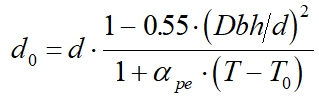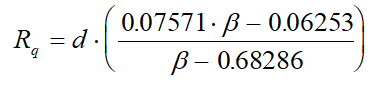Calculation of Beta Ratio - Intergraph Smart Instrumentation - 13.1 - Reference - Hexagon PPM

Integraph Smart Instrumentation Sizing Equations

Language
English (United States)
Product
Intergraph Smart Instrumentation
Search by Category
Reference
Smart Instrumentation Version
13.1
1. Calculate internal pipe diameter at flow temperature: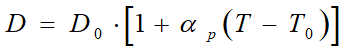2. Calculate correction factor for steam quality. (For liquid, water, and gas, Fs=1):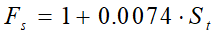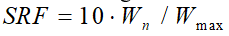4. Calculate differential pressure at normal flow rate: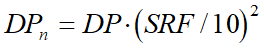5. Calculate Pipe Reynolds number at normal flow rate: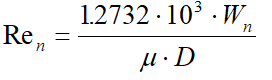6. Calculate first estimate for b ratio:

1. For Venturi tubes: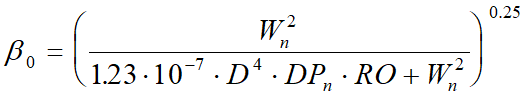2. For other Flow meters: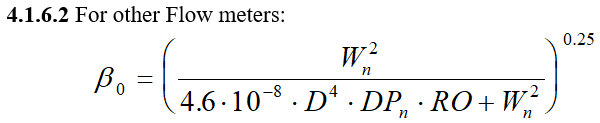7. Calculate gas expansion factor (for liquid and water, Eps = 1).

1. For square-edge orifice with 21/2D and 8D pipe taps: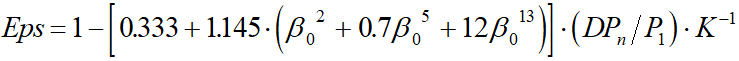2. For Lo-Loss tubes, Venturi tubes and nozzles: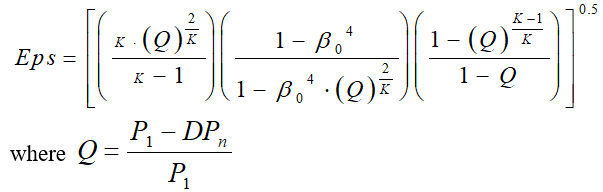3. For eccentric orifices: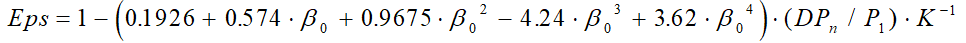4. For square-edge orifices specified in ISO 5167-2003 (flange tappings, corner tappings, and radius tappings):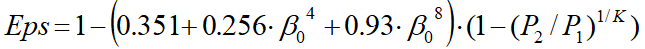5. For other Flow meters: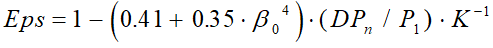9. Calculate new b - ratio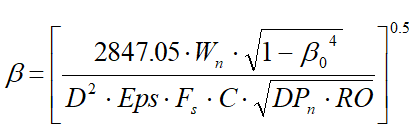10. Calculate the relative change in b - ratio.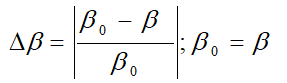11. Repeat steps 7 to 10 until the relative change in the b -ratio is less than 0.0001.

12. Calculate orifice diameter at flow temperature:

1. For all Flow meters except Segmental orifices: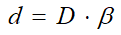2. Segmental orifices: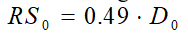2. Calculate Segmental height at flow temperature using the equation: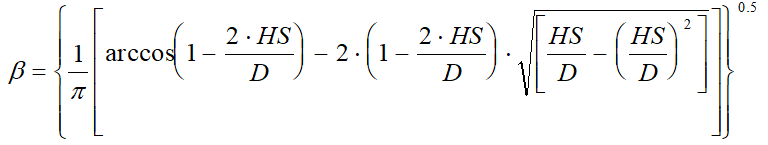For further calculation of Segmental orifices, HS and HS0 are analogous to d and d0 respectivly.

13. Calculate orifice diameter at ambient temperature:

1. For Lo-Loss tube, nozzles and Venturi tubes: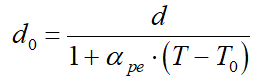2. For other orifices: